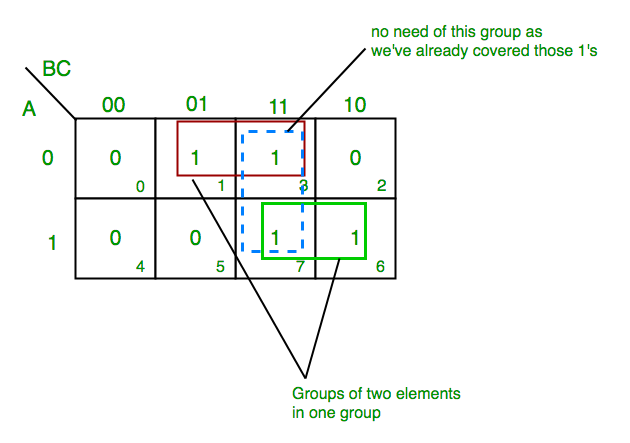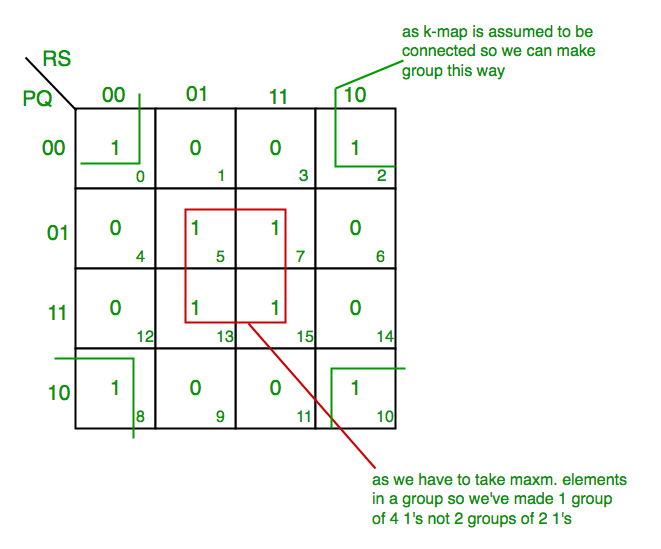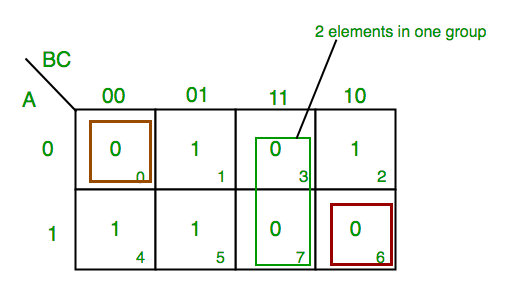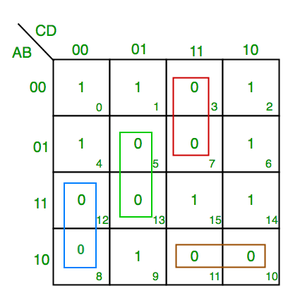# Introduction of K-Map (Karnaugh Map)

In many digital circuits and practical problems we need to find expression with minimum variables. We can minimize Boolean expressions of 3, 4 variables very easily using K-map without using any Boolean algebra theorems. K-map can take two forms Sum of Product (SOP) and Product of Sum (POS) according to the need of problem. K-map is table like representation but it gives more information than TRUTH TABLE. We fill grid of K-map with 0’s and 1’s then solve it by making groups.

Steps to solve expression using K-map-

1. Select K-map according to the number of variables.
2. Identify minterms or maxterms as given in problem.
3. For SOP put 1’s in blocks of K-map respective to the minterms (0’s elsewhere).
4. For POS put 0’s in blocks of K-map respective to the maxterms(1’s elsewhere).
5. Make rectangular groups containing total terms in power of two like 2,4,8 ..(except 1) and try to cover as many elements as you can in one group.
6. From the groups made in step 5 find the product terms and sum them up for SOP form.

## SOP FORM

1. K-map of 3 variables-

Z= ∑A,B,C(1,3,6,7)From red group we get product term—

A’C

From green group we get product term—

AB

Summing these product terms  we get- Final expression (A’C+AB)

1. K-map for 4 variables

F(P,Q,R,S)=∑(0,2,5,7,8,10,13,15)From red group we get product term—

QS

From green group we get product term—

Q’S’

Summing  these product terms  we get- Final expression (QS+Q’S’)

## POS FORM

1. K-map of 3 variables-

F(A,B,C)=π(0,3,6,7)From red group we find  terms

A    B      C’

Taking complement of these two

A’     B’     C

Now sum up them

(A’ + B’ + C)

From green group we find  terms

B         C

Taking complement of these two terms

B’         C’

Now sum up them

(B’+C’)

From brown group we find terms

A’ B’ C’

Taking complement of these two

A B C

Now sum up them

(A + B + C)

We will take product of these three terms :Final expression (A’ + B’ + C) (B’ + C’) (A + B + C)

2. K-map of  4 variables-

F(A,B,C,D)=π(3,5,7,8,10,11,12,13)From green group we find  terms

C’     D     B

Taking their complement and summing them

(C+D’+B’)

From red group we find terms

C     D    A’

Taking their complement and summing them

(C’+D’+A)

From blue  group we find  terms

A     C’     D’

Taking their complement and summing them

(A’+C+D)

From brown  group we find  terms

A    B’    C

Taking their complement and summing them

(A’+B+C’)

Finally we express these as product –(C+D’+B’).(C’+D’+A).(A’+C+D).(A’+B+C’)

PITFALL  *Always remember POS ≠ (SOP)’

*The correct form is (POS of F)=(SOP of F’)’

Attention reader! Don’t stop learning now. Get hold of all the important CS Theory concepts for SDE interviews with the CS Theory Course at a student-friendly price and become industry ready.

My Personal Notes arrow_drop_up

Improved By : pdabre18

Article Tags :

23

Please write to us at contribute@geeksforgeeks.org to report any issue with the above content.